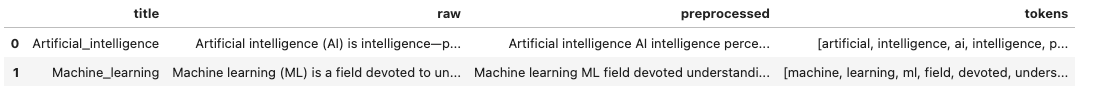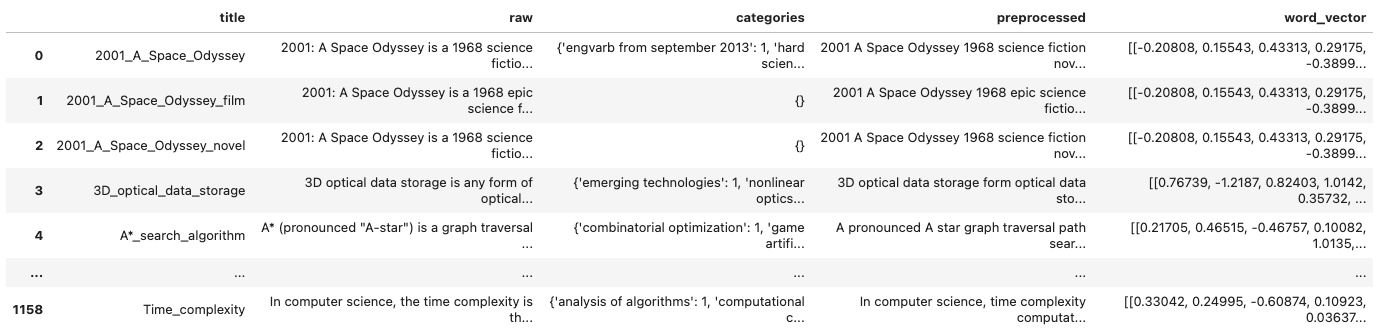# NLP Project: Text Vectorization Usage with Pandas and SciKit Learn

Posted in Nlp, Python, Word_vectors, Pandas

In any NLP project, text data needs to be vectorized in order to be used for machine learning algorithms. Different methods exist, starting from simple on-hot or count encodings, and continuing with term frequency metrics and word embeddings.

In my recent articles, text vectorization methods from scratch and SciKit learn included methods were explained and their application shown. The focus of this blog post is to apply the text vectorization methods for a KMeans clustering. During this application, I encountered a persistent error when trying to apply KMeans directly to preprocessed text or token lists stored in a Pands DataFrame. This error led to an in-depth study of the data structure differences between expected SciKit Learn input and Pandas DataFrame.

The technical context of this article is `Python v3.11`, `pandas v2.0.1` and `scikit-learn v1.2.2`. All examples should work with newer versions too.

## The Context

This article is part of a blog series about NLP with Python. In my previous articles, I covered how to create a Wikipedia article crawler, which will take an article name as the input, and then systematically downloads all linked articles until the given total number of articles or depth is achieved. Also, a corpus object abstracts individual articles and provides statistic like the number of sentences or paragraphs, as well as the vocabulary of all files.

For this article, the `WikipediaCorpus` object to crawled 200 pages starting with machine learning and artificial intelligence.

``````reader = WikipediaReader(dir = "articles")
``````

The articles are wrapped by a corpus object, and a SciKit Learn pipeline is created that will preprocess and tokenize the text, and create nested word vectors.

``````corpus = WikipediaPlaintextCorpus('articles')
corpus.describe()
#{'files': 189, 'paras': 15039, 'sents': 36497, 'words': 725081, 'vocab': 32633, 'max_words': 16053, 'time': 4.919918775558472}

pipeline = Pipeline([
('corpus', WikipediaCorpusTransformer(root_path='./articles')),
('preprocess', TextPreprocessor(root_path='./articles')),
('tokenizer', TextTokenizer()),
('word_vectorizer', WordVectorizer())
])
``````

The resulting data frame looks as follows:## The Problem

A simple KMeans clustering algorithm trying to identify 5 clusters in the data is created as follows:

``````model = KMeans(n_clusters=5, random_state=0, n_init="auto")
model.fit_transform(df['word_vector'])
``````

However, I encountered the following error message when using the data frame directly as the input:

``````---------------------------------------------------------------------------
TypeError                                 Traceback (most recent call last)
TypeError: float() argument must be a string or a real number, not 'list'

The above exception was the direct cause of the following exception:

ValueError                                Traceback (most recent call last)
Cell In, line 2
1 model = KMeans(n_clusters=2, random_state=0, n_init="auto")
----> 2 model.fit_transform(X['word_vector'])
...
File ~/Library/Caches/pypoetry/virtualenvs/nltk-jupyter-notebook-AZshehDx-py3.11/lib/python3.11/site-packages/pandas/core/series.py:917, in Series.__array__(self, dtype)
870 """
871 Return the values as a NumPy array.
872
(...)
914       dtype='datetime64[ns]')
915 """
916 values = self._values
--> 917 arr = np.asarray(values, dtype=dtype)
918 if using_copy_on_write() and astype_is_view(values.dtype, arr.dtype):
919     arr = arr.view()

ValueError: setting an array element with a sequence.
``````

This error persisted even when I used other vectorization methods that stored their values directly in the Pandas DataFrame and when this was used as an input to the SciKit Learn.

## Troubleshooting Options

To understand the origin of, and solution to, this processing problem, following investigation stages were performed:

1. Apply Built-In Vectorization Method
2. Use Word Vectors from DataFrame
3. Apply Dimensionality Reduction

Each of these options is explained in the following sections.

## Try 1: Apply Built-In Vectorization Method

The first attempt is to use the build-in `DictVectorizer`. For this, a small helper method is required to produce a bag-of-word representation of the tokenized text, and then apply the vectorizer to it.

``````from collections import Counter

def bag_of_words(tokens):
return Counter(tokens)

X['bow'] = X['tokens'].apply(lambda tokens: bag_of_words(tokens))
``````

Then the vectorizer is applied:

``````from sklearn.feature_extraction import DictVectorizer

X_dict_vector = DictVectorizer().fit_transform(X_prep['bow']).to_array()
``````

Investigating the shape and the type of the resulting object yields the following results:

``````print(X_dict_vector)
# [[ 89.   0.   5. ...   0.   0.   0.]
#  [ 56.   0.  13. ...   0.   0.   0.]
# [  2.   0.   4. ...   0.   0.   0.]
#  ...
#  [554.   0. 373. ...   0.   0.   0.]
#  [104.   0.  81. ...   0.   0.   0.]
#  [ 57.   0.  46. ...   0.   0.   0.]]

print(type(X_dict_vector))
# numpy.ndarray

print((X_dict_vector).shape)
# (195, 35940)

print(type(X_dict_vector))
# numpy.ndarray

print((X_dict_vector).shape)
# (35940,)
``````

This representation can be used as K-Means input.

``````model = KMeans(n_clusters=2, random_state=0, n_init="auto")

model.fit_transform(X_dict_vector)
# array([[ 98.75735089, 460.26180189],
#       [ 82.42298588, 474.97876188],
#       [121.74175722, 522.31041649],
#       [ 96.49885001, 493.65329872],
#       [305.0252639 , 259.96688909],
``````

However, I can not store it in the Pandas dataframe.

``````X['bow_dict'] = DictVectorizer().fit_transform(X['bow']).todense()

# ValueError: Expected a 1D array, got an array with shape (189, 34872)
``````

Is this data structure difference - the format stored/required to be stored in a data frame, vs. the sparse matrix representation - the root problem?

## Try 2: Use Word Vectors from DataFrame

Based on this, lets investigate the structure of the word vectors stored in the data frame and understand why they cannot be used as well.

``````X_word_vector = X['word_vector']

print(X_word_vector)
#[array([-0.20808 ,  0.15543 ,  0.43313 ,  0.29175 , -0.38998 ,  0.2216  ,
#       -0.96475 , -0.57093 ,  0.089177,  0.03206 ,  0.20457 , -0.55757 ,
#       -0.99732 , -0.30656 ,  1.34    , -0.19643 , -0.56457 ,  0.013656,
#       -1.055   ,  0.81931 ,  0.49625 , -0.21102 ,  0.56594 , -0.27317 ,

print(type(X_word_vector))
#pandas.core.arrays.numpy_.PandasArray

print(type(X_word_vector))
#list
``````

Ok! The content of the word vectors are stored as lists, not as arrays. We need to convert this.

``````X['word_vector_conv'] = X['word_vector'].apply(lambda vec: np.array(vec, dtype=np.float64))
X_word_vector=X['word_vector_conv']
``````

Lets investigate this too

``````print(type(X_word_vector))
#<class 'pandas.core.series.Series'>

print(type(X_word_vector))
#numpy.ndarray

print(type(X_word_vector))
#numpy.ndarray

print((X_word_vector))
# [[-0.20807999  0.15543     0.43313    ... -0.17567     0.022405
#  -0.19712   ]
# [ 0.21705     0.46515    -0.46757001 ... -0.043782    0.41012999
``````

Lets try if KMeans clustering can work with this data structure:

``````model = KMeans(n_clusters=2, random_state=0, n_init="auto")

model.fit(X_word_vector)
# ....
# ValueError: setting an array element with a sequence.
``````

No, that’s not enough. Although we now use only `ndarrays`, the nested structure is not parseable. We need to flatten and reduce the array of array to a single array. This calls for dimensionality reduction.

## Try 3: Apply Dimensionality Reduction

The 50-dimensional word vectors create huge data structure for articles with 3000 words. Reducing these high dimensional values improves downstream processing for clustering algorithms. Especially in the context of KMeans, the principal component analysis, or short `PCA` often used. Its application is made easy with a very streamlined API: Just define the number of components to keep.

Here is the declaration and application of PCA to the word vector list.

``````from sklearn.decomposition import PCA

pca = PCA(n_components=20)

def pca_reduce(vec_list):
vec = np.array(vec_list, dtype=np.float64)
vec = pca.fit_transform(vec)
return vec.flatten()

X['pca'] = X['word_vector'].apply(lambda vec_list: pca_reduce(vec_list))
``````

The resulting shape is as follows:

``````X_pca = X['pca']

print(X_pca.shape)
# (3700,)

print(type(X_pca))
#numpy.ndarray>

print(type(X_pca))
#numpy.float64

print((X_pca))
#[-0.37691919  0.25146476 -0.62295832 ...  0.12095541 -0.131852
#  0.69806209]
``````

However, resulting vectors still have different lengths:

``````print(X_pca.shape)
# (3700,)

print(X_pca.shape)
#(174820,)

print(X_pca.shape)
#(1340,)
``````

And can not be used with KMeans, we need to provide padding to these values as well.

## Try 4: Padding Word Vectors

For the KMeans clustering, numerical input data needs to be of the same length. For variable length text documents, this actually means that we need to apply both padding, which is filling the vector with additional tokens, and cutting all of the remaining tokens.

Numpy provides the `np.pad` function for this feature, which is used in the following modified pca method:

``````def pca_reduce_with_padding(vec_list,padding):
vec = np.array(vec_list, dtype=np.float64)
vec = pca.fit_transform(vec)
return vec.flatten()[:100000]

pca = PCA(n_components=20)

X['pca'] = X['word_vector'].apply(lambda vec_list: pca_reduce_with_padding(vec_list, 100000))
``````

Let’s investigate the type and shape of the data.

``````print(type(X['pca']))
# pandas.core.series.Series
print(X['pca'].shape)
# (200,)

print(type(X['pca']))
# <class 'numpy.ndarray'>
print(X['pca'].shape)
# (5000,)
print(X['pca'])
#[-0.37691919  0.25146476 -0.62295832 ...  0.          0.
#  0.        ]

print(X['pca'].shape)
# (50100000,)
print(X['pca'])
#[-0.84294759  0.97485764  1.55838785 ... -0.60164471  0.61974612
# -0.21179691]
``````

The word vectors are stored in the DataFrame.This looks good. Now, as we learned from applying the `DictVectorizer`, we cannot apply KMens to the data stored in the DataFrame but need to convert it to a matrix representation.

Here is a small helper method:

``````def to_matrix(series):
return np.stack(series.values)

x = to_matrix(X['pca'])

print(x.shape)
# (200, 5000)
``````

And finally, we can use this data with KMeans clustering.

``````model = KMeans(n_clusters=2, random_state=0, n_init="auto")

model.fit_transform(x)
#array([[46.65559992, 45.98162989],
#       [55.00747482, 54.44194782],
#       [54.91935663, 53.87752277],
#       [56.90356272, 56.20664703],
#       [56.78261868, 56.07188597],
#       [55.66976216, 55.27883963],
#       [58.50760043, 59.44522665],

model.labels_
#array([1, 1, 1, 1, 1, 1, 0, 0, 0, 0, 1, 0, 1, 1, 1, 1, 0, 1, 0, 1, 1, 1,
#       0, 0, 1, 1, 1, 0, 1, 1, 1, 1, 0, 1, 1, 1, 1, 1, 1, 1, 0, 0, 1, 1,
#       1, 0, 0, 0, 0, 1, 1, 1, 0, 1, 1, 1, 1, 0, 0, 0, 1, 0, 0, 0, 0, 1,
#       1, 0, 0, 1, 1, 1, 1, 0, 1, 0, 0, 0, 0, 0, 1, 1, 1, 1, 1, 1, 0, 0,
#       1, 0, 1, 0, 0, 0, 1, 1, 0, 0, 1,
``````

## Conclusion

This article investigated how to transform a Pandas DataFrame object representing word vectors to a Numpy array suitable for applying the clustering algorithms. In essence, DataFrame object do not work as-is with SciKit Learn, because selecting them yields a `Series` object, and its column value for array-like structures is `PandasArray`. The first step is to transform them to Numpy arrays, and the second step is to ensure that the data shape is `number_of_samples, vector_data`, where vector needs to be a flat array. To arrive at this structure, the word-vector representation was reduced from 50 dimensions to 20 using PCA, then the vectors were padded and truncated. This structure was saved in a DataFrame, and then manually converted to a Numpy matrix that could be used for input to the clustering algorithm.